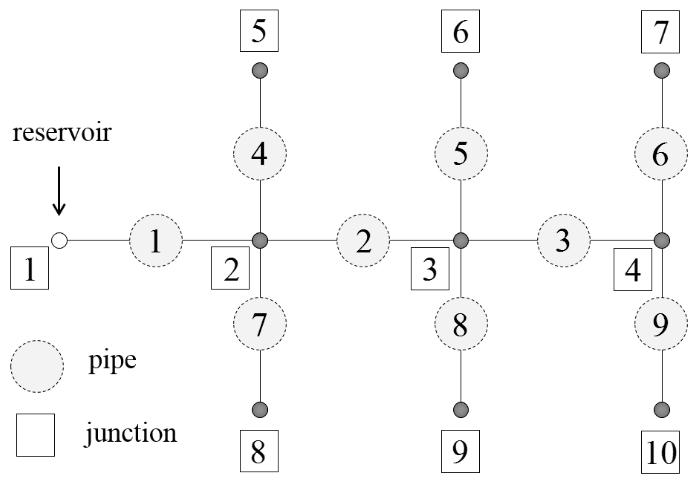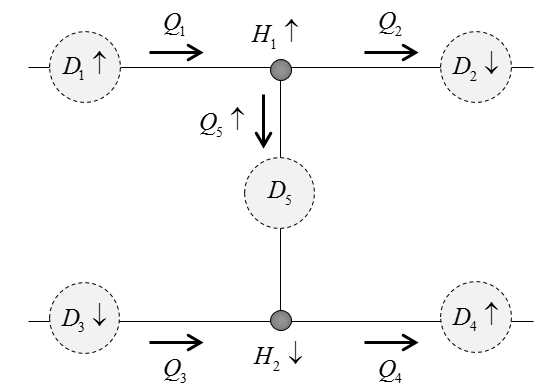Stipanić, D., Travaš, V., Kranjčević, L., Holjević, D.

An Iterative Algorithm for Optimizing Pipe Diameter in Pressurized System
Technical Gazette, (2020), doi.org/10.17559/TV-20190305122027, (quartile Q4)

Abstract: In order to achieve optimal flow velocity in stationary flow condition in pressurized pipe systems, it is necessary to determine an optimal value of pipe diameter. It should be noted that even for a simple pipe network with pipes connected in only few numbers of loops, the determination of the optimal pipe diameter is an unintuitive problem and from the mathematical point of view a nonlinear problem that requires some iterative process whit a relatively complicated update procedure between iterations. Usually, the optimization algorithms are based on stochastic methods. A deterministic approach is proposed that satisfy the prescribed constrains in terms of the specified flow velocity in the pressurized system of pipes. The presented iterative algorithm for optimizing the pipe diameter is implemented in computer code PIPENET3D (written in FORTRAN90) and used for hydraulic analysis of steady flow in an arbitrary pressured pipe system.